Definition of Sublevel

What is a Sublevel?

A sublevel is an energy level defined by quantum theory. In chemistry, sublevels refer to energies associated with electrons. In physics, sublevels may also refer to energies associated with the nucleus.

Niels Bohr's earliest quantum theory said that electrons occupy spherical shells centered on the atomic nucleus, such as the two electron shells shown for lithium below: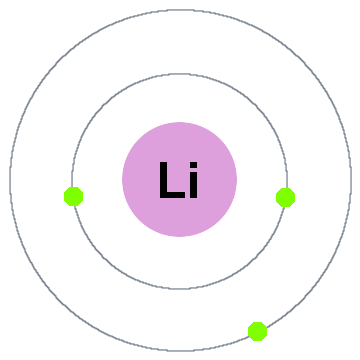In this old shell model moving outward:

• Shell 1 can hold up to 2 electrons,
• Shell 2 can hold up to 8 electrons,
• Shell 3 can hold up to 18 electrons,
• Shell 4 can hold up to 32 electrons,
• Shell 5 can hold up to 50 electrons,...

Each shell is actually an energy level. The higher the shell, the higher the energy of its electron(s). All the electrons sharing a shell are degenerate, meaning they have the same amount of energy.

Splitting the Shells into Subshells

Advances in spectroscopy revealed that shells can actually contain subshells/sublevels. The electrons in shell 3, for example, might have different amounts of energy from one another because they occupy different sublevels.

The energy level diagram below shows sublevels to as high as the energy level of the 5f orbitals. Sublevels actually continue to higher energies than this, but 5f is a suitable place to leave an introductory description.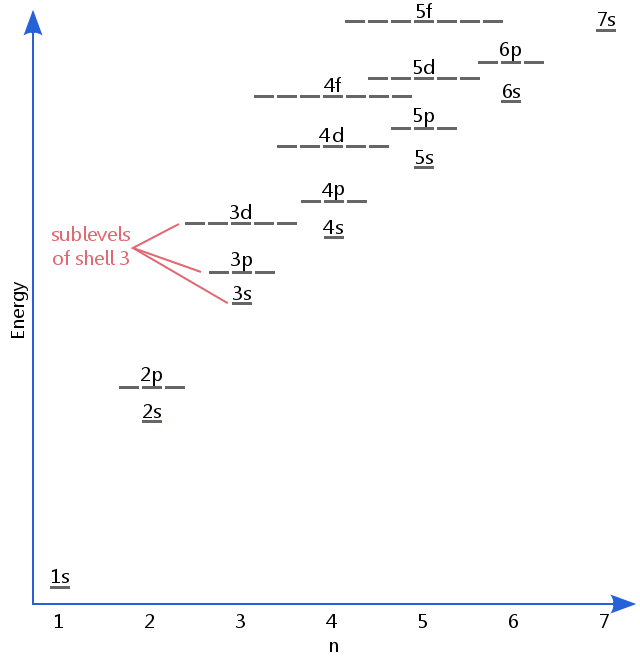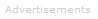Naming the Subshells/Sublevels

Electron sublevels are known by the letters s, p, d, and f. So, for example, electrons in the s sublevel of shell 3 have a different amount of energy from electrons in the p and d levels of shell 3. (This is not the case for hydrogen. All of hydrogen's sublevels have the same energy, because hydrogen only has one electron.)

s, p, d, and f sublevels

The sublevel occupied by any electron is determined by the electron's angular momentum quantum number, l.

Angular Momentum Quantum Number and Sublevel Type

Angular Momentum Quantum Number, lSublevelNumber of electrons sublevel can accommodate
0 s 2
1 p 6
2 d 10
3 f 14

The energy level diagram excerpt below shows the sublevels corresponding to different values of l in the fourth electron shell.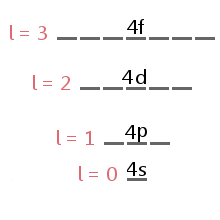Sublevel orbitals have characteristic shapes that can be used to explain and predict the chemical bonds that atoms can form. These shapes are based on the likelihood of finding an electron at any particular location orbiting the nucleus.

For example, we can say that an electron in a hydrogen atom's 1s sublevel will be found 99 percent of the time somewhere in a sphere with a given radius around the nucleus. This is the reason we can draw the s sublevel's orbitals as a sphere.

Orbitals for higher sublevels are also drawn on the basis of where an atom's electrons are most likely to be found. See orbitals for more details.

An s sublevel's orbital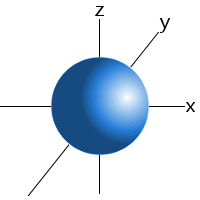Sublevel Examples
Examples of the sublevels found in various atoms are shown below. The superscript shows the number of electrons in each sublevel.

Hydrogen: 1s1

Carbon: 1s2 2s2 2p2

Chlorine: 1s2 2s2 2p6 3s2 3p5

Argon: 1s2 2s2 2p6 3s2 3p6

In general, electrons go into the lowest available energy sublevel. The general order in which sublevels are filled is:

1s 2s 2p 3s 3p 4s 3d 4p 5s 4d 5p 6s 4f 5d 6p 7s 5f 6d 7p

Given this, we can answer questions like: "what are potassium's electron sublevels?"

Potassium has 19 electrons, and we know that s orbitals hold a maximum of two electrons and p a maximum of six. Potassium's electron sublevels will be:

1s2 2s2 2p6 3s2 3p6 4s1

For some individual atoms with more than 20 electrons the 3d orbitals may fill before the 4s orbitals. Also, there may be other exceptions to this filling order, as discussed in the comments section for iodine.Search the Dictionary for More Terms